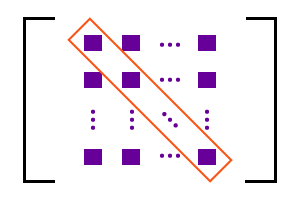# Principal Diagonal of a matrix

The straight path from first element of first row to last element of last row of a square matrix is called principal diagonal of the matrix.Leading diagonal of Matrix

The principal diagonal of a square matrix is also called as leading diagonal of the matrix. A straight path can be formed by joining the two elements which are at two opposite corners of a square matrix. One corner is position of first element of first row and another corner is position of last element of last row. The diagonal which joins them contains some elements in the case of square matrices which are higher than second order square matrix.

$M=\left[\begin{array}{ccccc}{e}_{1⁣1}& {e}_{1⁣2}& {e}_{1⁣3}& \cdots & {e}_{1⁣n}\\ {e}_{2⁣1}& {e}_{2⁣2}& {e}_{2⁣3}& \cdots & {e}_{2⁣n}\\ {e}_{3⁣1}& {e}_{3⁣2}& {e}_{3⁣3}& \cdots & {e}_{3⁣n}\\ ⋮& ⋮& ⋮& \ddots & ⋮\\ {e}_{n⁣1}& {e}_{n⁣2}& {e}_{n⁣3}& \cdots & {e}_{n⁣n}\end{array}\right]$

It is a square matrix, expressed in standard form. Consider the position of first element of first row and the element at that position is ${e}_{11}$ and also consider the position of last element of last row and the element at that position is ${e}_{nn}$. They both are at opposite corners and form a straight path by joining them and it is called principal diagonal or leading diagonal of a matrix.

Every element which is at a row with same column lies on the principal diagonal of the matrix. In other words, ${e}_{11},{e}_{22},{e}_{33}$ and up to ${e}_{nn}$ lie on the leading diagonal of square matrix.

## Example

$A$ is a square matrix of order $4×4$. It is having $16$ elements.

$A=\left[\begin{array}{cccc}1& 8& 0& 7\\ 6& 2& 8& 9\\ 5& 7& 3& 9\\ 6& 8& 5& 4\end{array}\right]$

$1$ is the first element of first row and $4$ is the last element of last row. The straight path that joins them is known as principal diagonal of matrix $A$. The leading diagonal also contains the elements $2$ and $3$. Therefore, the straight path which holds the elements $1,2,3$ and $4$ is leading diagonal of matrix A

Email subscription
Math Doubts is a best place to learn mathematics and from basics to advanced scientific level for students, teachers and researchers. Know more
Follow us on Social Media
###### Math Problems

Learn how to solve easy to difficult mathematics problems of all topics in various methods with step by step process and also maths questions for practising.

Learn more